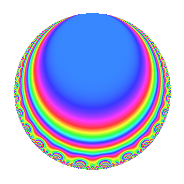# Properties

 Label 4032.2.v.aLevel 4032 Weight 2 Character orbit 4032.v Analytic conductor 32.196 Analytic rank 0 Dimension 4 CM No Inner twists 4

# Related objects

## Newspace parameters

 Level: $$N$$ = $$4032 = 2^{6} \cdot 3^{2} \cdot 7$$ Weight: $$k$$ = $$2$$ Character orbit: $$[\chi]$$ = 4032.v (of order $$4$$ and degree $$2$$)

## Newform invariants

 Self dual: No Analytic conductor: $$32.195682095$$ Analytic rank: $$0$$ Dimension: $$4$$ Relative dimension: $$2$$ over $$\Q(i)$$ Coefficient field: $$\Q(\zeta_{8})$$ Coefficient ring: $$\Z[a_1, \ldots, a_{25}]$$ Coefficient ring index: $$2$$ Sato-Tate group: $\mathrm{SU}(2)[C_{4}]$

## $q$-expansion

Coefficients of the $$q$$-expansion are expressed in terms of a basis $$1,\beta_1,\beta_2,\beta_3$$ for the coefficient ring described below. We also show the integral $$q$$-expansion of the trace form.

 $$f(q)$$ $$=$$ $$q$$ $$+ ( \beta_{2} + \beta_{3} ) q^{5}$$ $$- q^{7}$$ $$+O(q^{10})$$ $$q$$ $$+ ( \beta_{2} + \beta_{3} ) q^{5}$$ $$- q^{7}$$ $$+ ( -\beta_{2} + \beta_{3} ) q^{11}$$ $$+ ( 2 + 2 \beta_{1} ) q^{13}$$ $$-5 \beta_{2} q^{23}$$ $$-\beta_{1} q^{25}$$ $$+ ( \beta_{2} - \beta_{3} ) q^{29}$$ $$-8 \beta_{1} q^{31}$$ $$+ ( -\beta_{2} - \beta_{3} ) q^{35}$$ $$+ ( 5 - 5 \beta_{1} ) q^{37}$$ $$+ 2 \beta_{3} q^{41}$$ $$+ ( 3 + 3 \beta_{1} ) q^{43}$$ $$+ 4 \beta_{3} q^{47}$$ $$+ q^{49}$$ $$+ 4 q^{55}$$ $$+ ( 2 \beta_{2} - 2 \beta_{3} ) q^{59}$$ $$+ ( -2 - 2 \beta_{1} ) q^{61}$$ $$+ 4 \beta_{2} q^{65}$$ $$+ ( 5 - 5 \beta_{1} ) q^{67}$$ $$+ 7 \beta_{2} q^{71}$$ $$+ 14 \beta_{1} q^{73}$$ $$+ ( \beta_{2} - \beta_{3} ) q^{77}$$ $$-16 \beta_{1} q^{79}$$ $$+ ( -2 \beta_{2} - 2 \beta_{3} ) q^{83}$$ $$+ 8 \beta_{3} q^{89}$$ $$+ ( -2 - 2 \beta_{1} ) q^{91}$$ $$-6 q^{97}$$ $$+O(q^{100})$$ $$\operatorname{Tr}(f)(q)$$ $$=$$ $$4q$$ $$\mathstrut -\mathstrut 4q^{7}$$ $$\mathstrut +\mathstrut O(q^{10})$$ $$4q$$ $$\mathstrut -\mathstrut 4q^{7}$$ $$\mathstrut +\mathstrut 8q^{13}$$ $$\mathstrut +\mathstrut 20q^{37}$$ $$\mathstrut +\mathstrut 12q^{43}$$ $$\mathstrut +\mathstrut 4q^{49}$$ $$\mathstrut +\mathstrut 16q^{55}$$ $$\mathstrut -\mathstrut 8q^{61}$$ $$\mathstrut +\mathstrut 20q^{67}$$ $$\mathstrut -\mathstrut 8q^{91}$$ $$\mathstrut -\mathstrut 24q^{97}$$ $$\mathstrut +\mathstrut O(q^{100})$$

Basis of coefficient ring:

 $$\beta_{0}$$ $$=$$ $$1$$ $$\beta_{1}$$ $$=$$ $$\zeta_{8}^{2}$$ $$\beta_{2}$$ $$=$$ $$\zeta_{8}^{3} + \zeta_{8}$$ $$\beta_{3}$$ $$=$$ $$-\zeta_{8}^{3} + \zeta_{8}$$
 $$1$$ $$=$$ $$\beta_0$$ $$\zeta_{8}$$ $$=$$ $$($$$$\beta_{3}\mathstrut +\mathstrut$$ $$\beta_{2}$$$$)/2$$ $$\zeta_{8}^{2}$$ $$=$$ $$\beta_{1}$$ $$\zeta_{8}^{3}$$ $$=$$ $$($$$$-$$$$\beta_{3}\mathstrut +\mathstrut$$ $$\beta_{2}$$$$)/2$$

## Character Values

We give the values of $$\chi$$ on generators for $$\left(\mathbb{Z}/4032\mathbb{Z}\right)^\times$$.

 $$n$$ $$127$$ $$577$$ $$1793$$ $$3781$$ $$\chi(n)$$ $$-1$$ $$1$$ $$-1$$ $$-\beta_{1}$$

## Embeddings

For each embedding $$\iota_m$$ of the coefficient field, the values $$\iota_m(a_n)$$ are shown below.

For more information on an embedded modular form you can click on its label.

Label $$\iota_m(\nu)$$ $$a_{2}$$ $$a_{3}$$ $$a_{4}$$ $$a_{5}$$ $$a_{6}$$ $$a_{7}$$ $$a_{8}$$ $$a_{9}$$ $$a_{10}$$
1583.1
 −0.707107 − 0.707107i 0.707107 + 0.707107i −0.707107 + 0.707107i 0.707107 − 0.707107i
0 0 0 −1.41421 1.41421i 0 −1.00000 0 0 0
1583.2 0 0 0 1.41421 + 1.41421i 0 −1.00000 0 0 0
3599.1 0 0 0 −1.41421 + 1.41421i 0 −1.00000 0 0 0
3599.2 0 0 0 1.41421 1.41421i 0 −1.00000 0 0 0
 $$n$$: e.g. 2-40 or 990-1000 Significant digits: Format: Complex embeddings Normalized embeddings Satake parameters Satake angles

## Inner twists

Char. orbit Parity Mult. Self Twist Proved
1.a Even 1 trivial yes
3.b Odd 1 yes
16.f Odd 1 yes
48.k Even 1 yes

## Hecke kernels

This newform can be constructed as the intersection of the kernels of the following linear operators acting on $$S_{2}^{\mathrm{new}}(4032, [\chi])$$:

 $$T_{5}^{4}$$ $$\mathstrut +\mathstrut 16$$ $$T_{11}^{4}$$ $$\mathstrut +\mathstrut 16$$